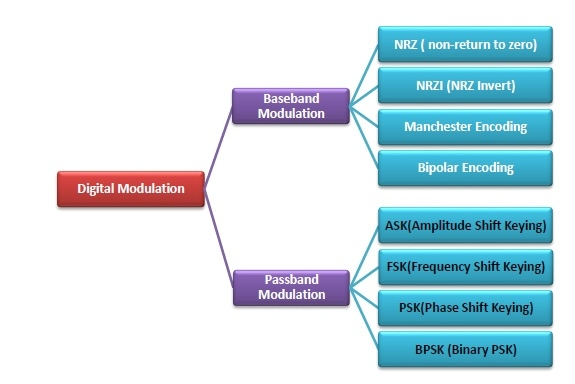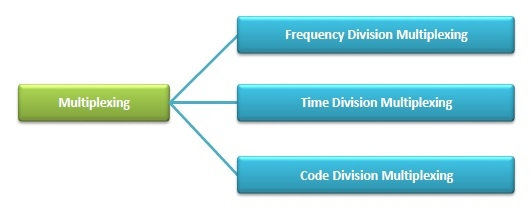# The Modulation and Multiplexing

Computer EngineeringComputer NetworkMCA

## Modulation

Modulation is the process of transforming a carrier signal so that it can carry the information of a message signal. It superimposes the contents of the message signal over a high-frequency carrier signal, which is then transmitted over communication channels.

Modulation can be of two types −

• Analog Modulation
• Digital Modulation

## Analog Modulation

Here, the analog information signal is transformed to the analog carrier signal so that it can travel large distances without substantial loss.

Analog modulation can be of three types −

• Amplitude Modulation
• Frequency Modulation
• Phase Modulation

## Digital Modulation

Digital modulation is the process of converting a digital bit stream into an analog carrier wave for transmission via a communication channel.

Digital modulation is broadly divided into two categories −

• Bandpass Modulation as in baseband transmission:

Here, the bits are converted directly into signals.

• Passband Modulation as in passband transmission:

Here, the amplitude, phase or frequency of the carrier signal is regulated to transmit the bits.

The following diagram illustrates the different digital modulation schemes −## Multiplexing

This is a method of combining more than one signal over a shared medium. Multiplexing divides the capacity of a communication channel into several logical channels, each for a data stream. The method of extracting the original data streams from the multiplexed signal is called demultiplexing.

The methods of multiplexing are as follows −